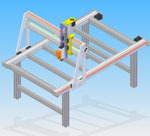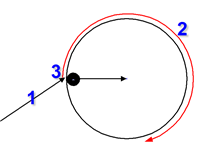Projecten

nul insteller

## Milling holes and circles

Van deze pagina is geen Nederlandse versie beschikbaar, hieronder staat de Engelstalige pagina.

Milling holes and circles in G-codeIt's not as difficult as it seems. G-code is a simple language and creating your own code is just a matter of logical thinking and some basic math. The picture at the left shows how to mill a large hole using a small mill. Remember, the mill rotates in a clockwise direction so if we are cutting a hole out of a sheet or a block, we need to make sure we mill in the right direction - which is also clockwise. Moving counterclockwise would be pulling the cutter out of the work piece and results in a bad result.

Now let's get going with some G-code. Milling a circle or an arc needs three sets of coordinates:

1. a starting point
2. a centre point
3. the end point

The mill now moves from point 1 to 3 around the centre point 3. If the start and end-point are the same a complete circle is made.
A circle always has to be located in a specific plane being either the XY, YZ or XZ plane and since I mostly just create 2.5D objects I am always milling circles in the XY plane.

To mill a hole (or actually only the contour of the hole, we need to take a number of steps:move to the starting point and place the mill at the required depth create a full circle by specifying the current point as endpoint and the centre coordinates as delta from the current point move the mill up, away from the workpiece

So to mill a circle with a radius of 4 and the centre at x,y = 10,10 at a depth of -1 we have to move to a starting point at the arc first, I take the given point in the picture above as starting point, this is as the same Y coordinate as the centre and the X coordinate is at x - radius (in this case 10-4 = 6). A move is always done in a straight line so only after arriving at the starting point we move down to -1. This is done using the following G-code:

```G0 x 6 y 10
G1 z -1```

A G0 (this is a zero, not the letter O) will move at high speed to the starting point and G1 will move the mill down at the feedrate (set with the F... command), for now I assume that the F speed is already set.

Now we are ready to move in a circle. We have to specify the XY plane (using G17) before starting to mill a clockwise circle (using G2). The centre location is specified relative from the starting point so for the center X equals to 4 (the radius) and Y 0. To mill a whole circle, we have to end at the same coordinates as we started from (so 6, 10):

`G17 G2 x 6 y 10 i 4 j 0 z -1`

As you can see there are two sets of coordinates specified in the line above: x, y specifies the end point and i, j the centre. I always specify the z coordinate also telling to stay at the same depth. Moving to a different Z-value would mill a helix (i.e. the mill would move up or down along the arc).

And clear away from the workpiece:

`G0 z 1`

So that's it, we are done - right?

No, all we did is move a full circle and if I had a 3mm flat tip cutter this will result in a hole that is too large. The centre of the cutter moves along the circle so we have removed too much material (on all sides the radius of the cutter) so we have to compensate for the cutter radius:

1. We have to start 1.5 mm further to the right from the starting point so we start at x = 6 + 1.5
2. The centre has to stay at the same location so the relative coordinate for the centre x is now 4 - 1.5

This results in the following piece of code:

```G0 x 7.5 y 10
G1 z -1
G17 G2 x 7.5 y 10 i 2.5 j 0 z -1
G0 z 1 ```

And now we are done! We have a contour that might leave a hole of the given size at the wanted location. If we need a hole with a large radius we need to repeat the movement starting with smaller circles and moving to larger ones until the wanted diameter is reached. Same goes for the depth, we can not remove too much material at once depending on the material, cutter type, spindle speed and feedrate.

Using variables

The example above is nice, but I do not want to calculate all this every time by hand. Variables in G-code are denoted by a # followed by a number. I could give a whole explanation of how to do this, but let's just show the code:

```#1 = 10        ( X of centre of circle )
#2 = 10        ( Y of centre )
#3 = 8         ( Diameter of circle )
#4 = 3         ( Cutter diameter )
#5 = -1        ( Z	depth )
#6 = [#3 - #4] ( Diameter of circle to mill
#6 = [#6 / 2]  ( Radius of circle to mill )

G0 z 2
G0 x [#1 - #6] y #2>
G1 z #5>
G17 G2 x [#1 - #6] y #2 i #6 j 0 z #5
G1 z 2```

First there are the five parameters to mill a hole at coordinates 10,10 at a depth of -1 with a radius of 4 (diameter = 8) and a 3mm cutter.
Then the milling circle is calculated, calculations on parameters always have to be within square brackets. The radius for that is (8-3)/2 = 2.5.

As you will notice I added an extra G0 z 2 at the beginning of the milling path, this is just to make sure that the movement towards the starting point is always done above the work piece. Otherwise there is a chance that we come across some pieces that are in between our current position and the starting point.
And the text in between brackets are just comments, these will be ignored by our milling centre.

Subroutines, using the same lines of G-code more than once

Ok, that worked. But now we want to create multiple circles without having to copy and paste all those lines of code through the whole file.
A subroutine is a piece of code that is only executed if we call that specific subroutine later on in the file. Subroutines (and looping constructs and decisions) are all marked with labels at the beginning and end of the section and the subroutine always starts with a label followed by sub and ends with a label with endsub.
All I know is that labels have numbers, maybe it is possible to use names but I have not seen the documentation to confirm this.

Parameters given to this subroutine when being called are also numbered, starting at #1 and ranging up to the number of parameters we specify in the call:

```( Mill a hole with the centre at x,y and diameter d, diameter of mill Md and depth z )
( Parameters:  x  y  d Md  z )
(             #1 #2 #3 #4 #5 )
O100 sub
#6 = [#3 - #4] ( Diameter of circle to mill )
#6 = [#6 / 2]  ( Radius of circle to mill )

G0 z 2
G0 x [#1 - #6] y #2
G1 z #5>
G17 G2 x [#1 - #6] y #2 i #6 j 0 z #5
G1 z 2
O100 endsub
```

And now we can call this subroutine as often as we like. So now we are done, milling our hole with a diameter of 8 mm can finally be completed.
Remember how we started with just milling the outer circle, leaving a bit in the middle because of the 3mm mill used? (I'll add some photos this weekend) By milling multiple circles with a diameter increase of no more than the diameter of the mill, we can mill the complete hole easily:

```O100 call     [-1]
O100 call     [-1]```

The first circle should always be smaller than twice the mill diameter, otherwise some material is left in the middle of the hole and on my machine I don't like to remove too much material at once so when milling edges (and the second circle is just a round edge) I never remove more than half the mill diameter in one go, so the circles are at max. the mill diameter apart.
This also depends of the material used, in foam I can cut away way more material (I did 2.5mm edges at 4mm deep) in one go but in Aluminium I am easing up a lot, mostly because I am still learning what the machine can handle and I don't have a decent cooling/lubricating system yet (I use a brush and do manual lubrication using oil)

The other way around: creating a round outer surface

Is easy to do. Just add the diameter of the mill to the diameter of the circle to mill and use a counterclockwise move using the G3 command instead of the G2.
I think you should be able to do that yourself by now.

To Do ...

I could post a complete .ngc file that mills a real object, e.g. the circuit board for my tool height indicator. That gives a nice example with just a bit more than only circles.